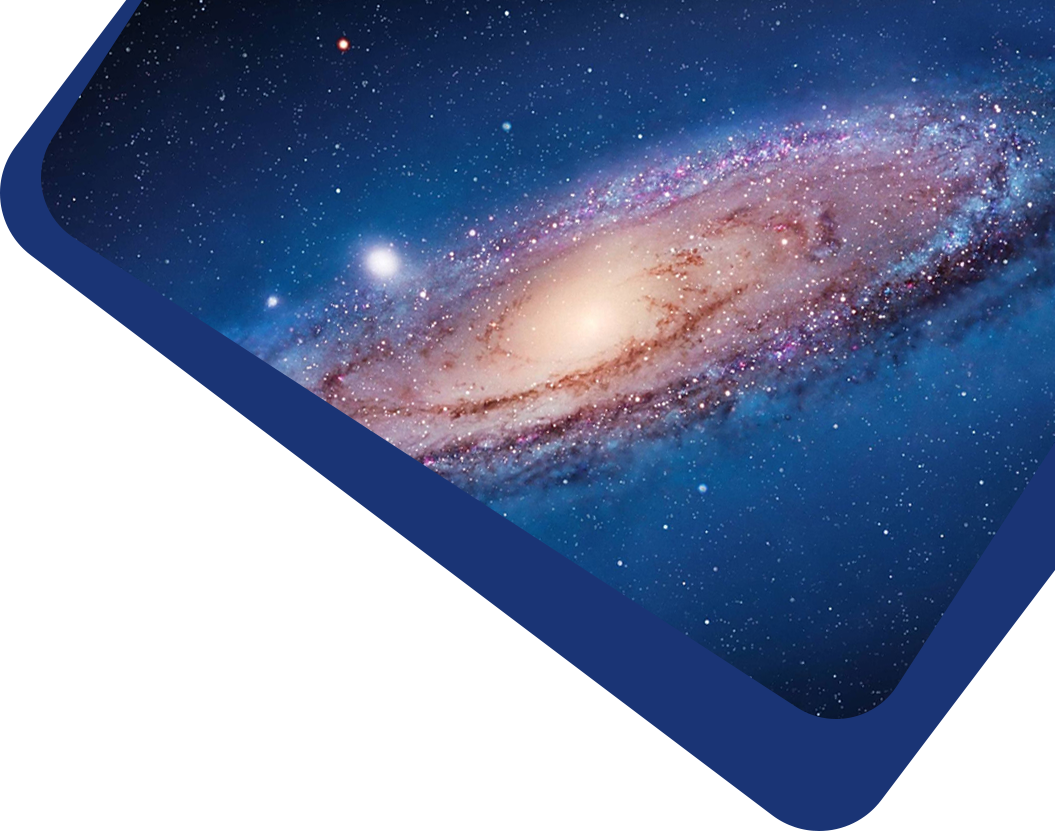Vol 20, No 9

## Investigating the relationship between cosmic curvature and dark energy models with the latest supernova sample

#### Chao Gao, Yun Chen, Jie Zheng

Abstract

Abstract We investigate the relationship between cosmic curvature and model of dark energy (hereafter DE) with recent Type Ia supernovae (hereafter SNe Ia) data, i.e., the Pantheon sample including 1048 SNe Ia with 0.01 < z < 2.3. We obtain measurements of the dimensionless spatial curvature density today, i.e., $$\Omega_{k0}=-0.062^{+0.189}_{-0.169}, -0.004^{+0.228}_{-0.134}, 0.127^{+0.280}_{-0.276}$$ and $$0.422^{0.213}_{-0.338}$$ at 68%confidence level (CL), respectively, in the scenarios of $$\Lambda$$CDM, $$\phi$$CDM (i.e., scalar field DE), $$\omega$$CDM and $$\omega_0\omega_a$$CDM models. In the scenario of $$\Lambda$$CDM model, a closed universe is preferred by the Pantheon sample, which is consistent with that from the Planck CMB spectra. However, the uncertainty of $$\Omega_{k0}$$ from the Pantheon SNe sample is about 8 times larger than that from the Planck data, so the former one supports a closed universe at a much lower CL than that from the latter one. An open unverse is supported by the Pantheon sample at ~32% and ~78% CLs, respectively, in the $$\omega$$CDM and $$\omega_0\omega_a$$CDM models. Among these models, the $$\phi$$CDM model is the one which supports the flat universe most strongly. It shows that $$\Omega_{k0}$$ is significantly dependent on the adopted model of DE, and there is a negative correlation between $$\Omega_{k0}$$ and the equation of state of DE.

Keywords

Keywords cosmological parameters—dark energy—cosmology: observations

Full Text
Refbacks

• There are currently no refbacks.